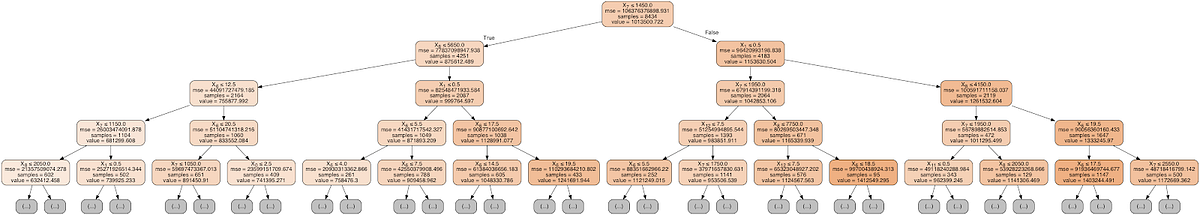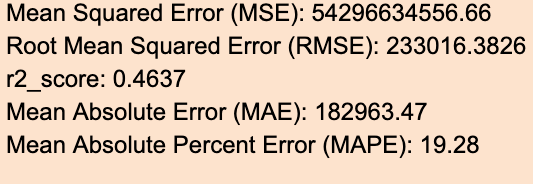# House Price Prediction in Natural Hazard Prone Areas - Part 2Different Regression models i.e. Linear Regression, Decision Tree Regression, Gradient Boosted Regression, and Random Forest Regression were used. The performance of those models using R² were compared. Based on these performance score, better performing model were suggested to predict house price.

## Introduction

Different Regression models i.e. Linear Regression, Decision Tree Regression, Gradient Boosted Regression, and Random Forest Regression were used. The performance of those models using R² were compared. Based on these performance score, better performing model were suggested to predict house price.

First, the data was divided into independent variable X and dependent variable y. Independent variable X was used to predict the target variable y. The price, id and date column were dropped from the new_df dataframe to create the variable X. The price column from the new_df dataframe were used to create the variable y. Different metrics were used to the performance of the Regression models such as Mean squared errors, Root mean squared errors, R-squared score, Mean absolute deviation, Mean absolute percent errors, etc. Root mean squared error and R-squared score were used to evaluate the performance of the regression model. In order to save the metrics of the model, a data frame was created and it was named metrics. Next, the data was splitted into training and testing set. 80% of the randomly selected data were kept as a training set and 20% of the randomly selected data as a testing set. The model was learned using the 80% of the data, and the rest 20% testing data were used as an unseen future dataset to predict the house price.

Linear Regression Model was built using the default parameters, and the model was fitted using the training dataset. X_test data was used to predict using the model. Then, Mean squared error (MSE), Root mean squared error (RMSE), R-squared score (r2_score), Mean absolute deviation (MAD), and Mean absolute percent error (MAPE) were calculated.## Feature Selection

Backward elimination method of feature selection were used. Feature selection is the process of selecting a subset of relevant features that may improve the performance of the model. First, the worst attribute from the feature was removed. The date_sold_month were removed because it has a very weak correlation with the price of the house. Then, year_built_decade_mapped were removed from the feature set. Then, a univariate feature selection package called SelectKbest from the sklearn library was tried. Below are correlation coefficients for different features.

## Use of Decision Trees and Random Forest in Machine Learning

Use of Decision Trees and Random Forest in Machine Learning. An Insight into Supervised Learning for Classification Problems

## Machine Learning: Random Forest, Adaboost & Decision Tree

Learn Advanced Machine Learning on Random Forest, Adaboost, Decision Trees Hands-on. Udemy Coupon Free

## Random forests — An ensemble of decision trees

This is how decision trees are combined to make a random forest. In this article, I describe how this can be used for a classification task with the popular Iris dataset.

## Decision Tree -Classification

Decision Tree is one of the most widely used machine learning algorithm. It is a supervised learning algorithm that can perform both classification and regression operations.

## Hire Machine Learning Developers in India

We supply you with world class machine learning experts / ML Developers with years of domain experience who can add more value to your business.